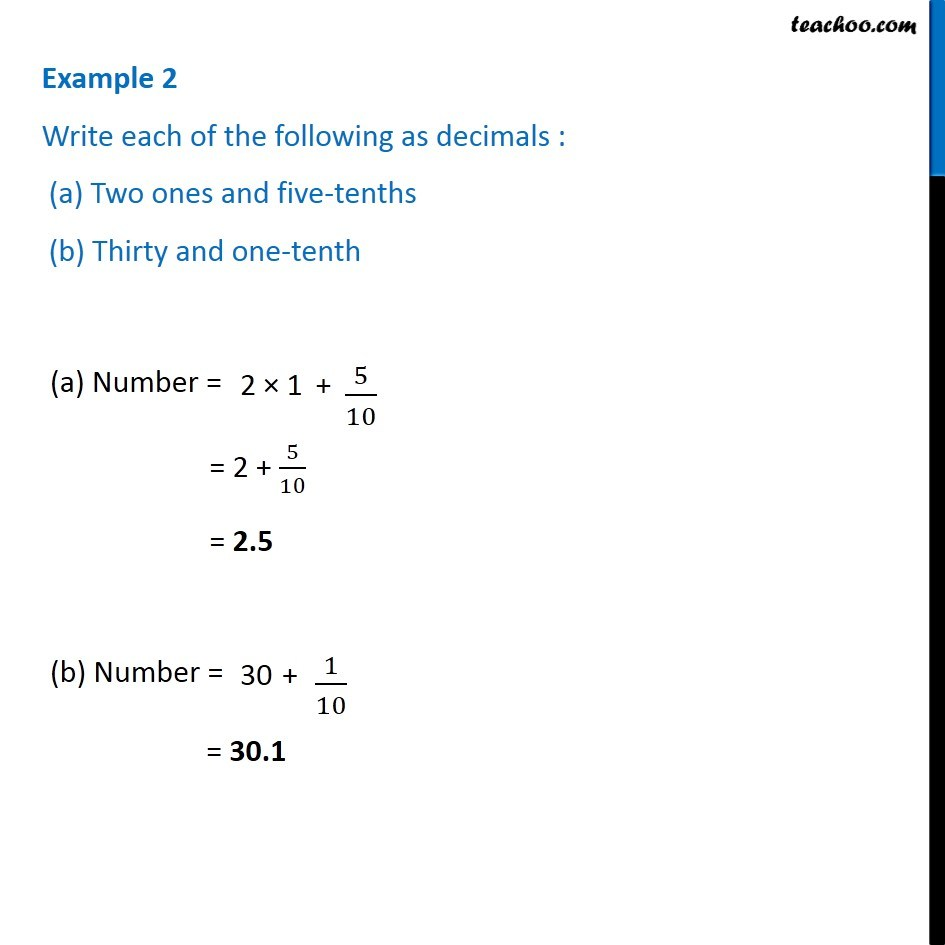Examples

Chapter 8 Class 6 Decimals
Serial order wiseGet live Maths 1-on-1 Classs - Class 6 to 12

### Transcript

Example 2 - Chapter 8 Class 6 Decimals Write each of the following as decimals : (a) Two ones and five-tenths (b) Thirty and one-tenth (a) Number = 2 x 1 + 5/10 = 2 + 5/10 = 2.5 (b) Number = 30 + 1/10 = 30.1